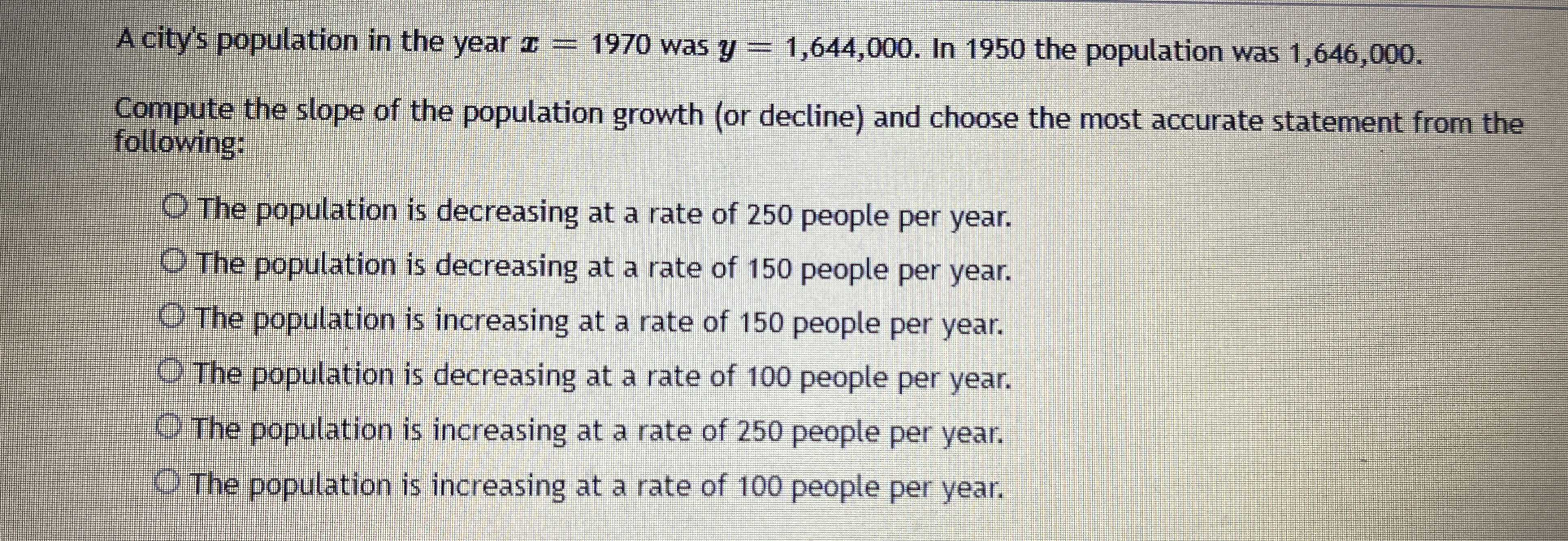### Still have math questions?

Algebra
QuestionA city's population in the year $$x = 1970$$ was $$y = 1,644,000 .$$ In $$1950$$ the population was $$1,646,000 .$$

Compute the slope of the population growth (or decline) and choose the most accurate statement from the following:

The population is decreasing at a rate of $$250$$ people per year.

The population is decreasing at a rate of $$150$$ people per year.

The population is increasing at a rate of $$150$$ people per year.

The population is decreasing at a rate of $$100$$ people per year.

The population is increasing at a rate of $$250$$ people per year.

The population is increasing at a rate of $$100$$ people per year.

The population is decreasing at a rate of $$100$$ people per year.